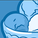# New to Qlik Sense

If you’re new to Qlik Sense, start with this Discussion Board and get up-to-speed quickly.

Announcements
Qlik Insider: SaaS capabilities for Data Integration and Analytics, May 25th! Register Now
cancel
Showing results for
Did you mean:Creator III

## Expressions equal to ''

Hello all, this is a random observation, but some of my metrics aren't running properly. Whenever I want to count something and am trying to see when a cell is equal to null, nothing returns. The data is there, but nothing shows up anymore ever since the new updates on Qlik Sense Cloud. Any other ideas to count when a column is equal to Null, followed is my expression.

(count({\$<[Dest. Handling Unit put]= {''}, [Warehouse Process Cat. Desc. put] = {'Putaway'}>} [Confirmed by put]))

+

(count({\$<[Dest. Handling Unit put]= {''}, [Warehouse Process Cat. Desc. put] = {'Internal Warehouse Movement'}>} [Confirmed by put])/2)

1 Solution

Accepted SolutionsCreator

Hi Joshua

One of the things I have trouble with in Qlik world is identifying nulls. It will show a null but not allow you to select one. Part of the problem is if your looking for a null in an associated table it seems to be different. I have some measures where the set analysis doesn't seem to work and where doing counts of what's missing i do a count of all minus where the value is populated.

So something like

(count({\$<[Warehouse Process Cat. Desc. put] = {'Putaway'}>} [Confirmed by put]))

-(count({\$<[Dest. Handling Unit put]-={''}, [Warehouse Process Cat. Desc. put] = {'Putaway'}>} [Confirmed by put]))

where the -={''} in the set analysis gives you the records where you have a value.

Also you can achieve OR logic in set analysis to avoid counting records that might satisfy both criteria if you have a need.

RonCreator

Hi Joshua

One of the things I have trouble with in Qlik world is identifying nulls. It will show a null but not allow you to select one. Part of the problem is if your looking for a null in an associated table it seems to be different. I have some measures where the set analysis doesn't seem to work and where doing counts of what's missing i do a count of all minus where the value is populated.

So something like

(count({\$<[Warehouse Process Cat. Desc. put] = {'Putaway'}>} [Confirmed by put]))

-(count({\$<[Dest. Handling Unit put]-={''}, [Warehouse Process Cat. Desc. put] = {'Putaway'}>} [Confirmed by put]))

where the -={''} in the set analysis gives you the records where you have a value.

Also you can achieve OR logic in set analysis to avoid counting records that might satisfy both criteria if you have a need.

Ron